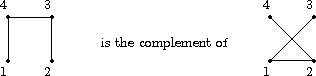Selfcomplementary graphs

### Selfcomplementary graphs

Two labelled graphs f, [~f] Î2 [v choose 2] are called complementary

if and only if

[~f] ( {i,j })=0 iff f( {i,j })=1.Two complementary labelled graphs

Correspondingly we say that two graphs, i.e. isomorphism classes of labelled ones, are complementary graphs if and only if one class arises from the other by forming the complements. The example of figure shows that graphs may very well be selfcomplementary , and hence we ask for the number of selfcomplementary graphs on v vertices. In order to prepare the derivation of this number, we notice that the classes which we obtain by putting a graph and its complement together in one class are just the orbits of the group H ´G :=S2 ´S v on the set YX:=2 [v choose 2](recall the section of paragigmatic examples). According to Theorem the number of these orbits is equal to

(1)/(2 ·v!) å( r, p) ÎS2 ´S v Õi=1[v choose 2] | 2 ri | ai( bar ( p)).

But | 2 ri | , the number of fixed points of ri, rÎS2, acting on the set 2, is either 2 or zero. Hence we separate the sum over the ( r, p) ÎS2 ´S v into two sums depending on rÎS2 and get the following expression for the number of these orbits:

| (S2 ´S v) \\2 [v choose 2] | =(1)/(2 ·v!) ( åp2c( bar ( p))+ åp' 2c( bar ( p)) ),

where åp means the sum over all the elements of Sv , while å'p means the sum over all the elements p of Sv such that bar ( p) does not contain a cycle of odd length, i.e. a2i+1( bar ( p))=0, for each i. There is a program to compute the number of orbits, which consist of graphs and their complements.

In order to derive from this equation the total number of selfcomplementary graphs on v vertices we use an easy argument which we have already met when we introduced the notions of enantiomeric pairs and selfenantiomeric orbits. A group of order 2 consisting of the identity map and the ''complementation `` acts on the set of graphs. This action is chiral if v >= 2, and hence the desired number of selfcomplementary graphs is twice the number given in examples minus the number in Corollary. We have obtained

Corollary: The number of selfcomplementary graphs on v vertices is equal to
(1)/(v!) åp'2c( bar ( p)) = åa'(2c bar (a) )/( Õiiaiai!) ,
where c bar (a) is as in Corollary, and where å'a denotes the sum over all the cycle types a such that for each element p with this cycle type, the corresponding bar ( p) does not contain any cycle of odd length.

There are some numerical results for the number of selfcomplementary graphs.

This leads to an existence theorem (cf. exercise):

Theorem: Selfcomplementary graphs on v vertices exist if and only if v is congruent to 0 or 1 modulo 4.

Proof: By corollary a selfcomplementary graph exists if and only if there are pÎS v such that bar ( p) does not contain any cycle of odd length. Now, if neither v º0 (4) nor v º1 (4), then [v choose 2] is odd so that each bar ( p), pÎS v, must contain a cyclic factor of odd length. On the other hand, if v º0 (4), then to the full cycle p:=(1 ...v) ÎS v there corresponds a permutation bar ( p) which, by lemma, does not contain a cyclic factor of odd length. Finally, in the case when v º1 (4), we consider p:=(1 ...v-1)(v). Again by lemma, bar ( p) does not contain any cyclic factor of odd length.

 harald.fripertinger "at" uni-graz.at http://www-ang.kfunigraz.ac.at/~fripert/ UNI-Graz Institut für Mathematik UNI-Bayreuth Lehrstuhl II für Mathematik
last changed: January 19, 2005Selfcomplementary graphs